Anúncio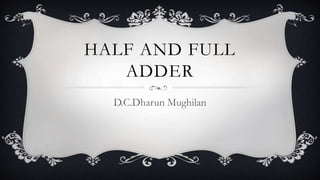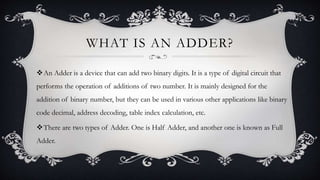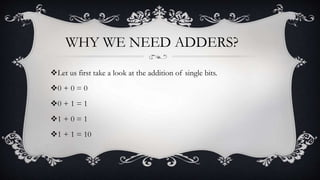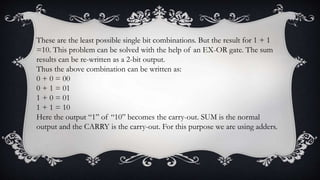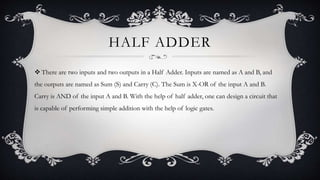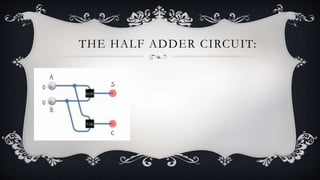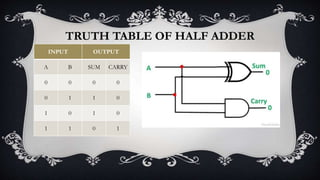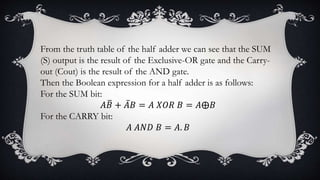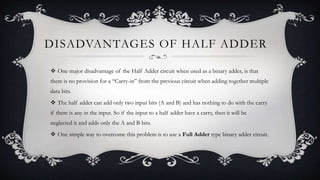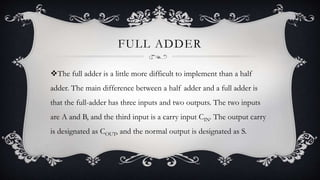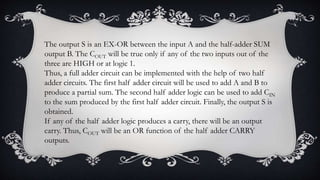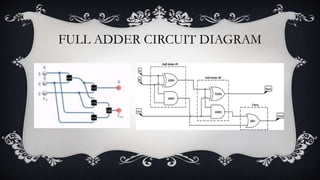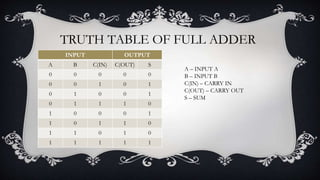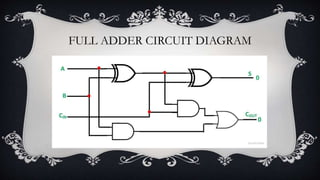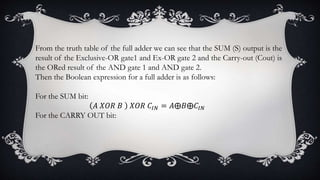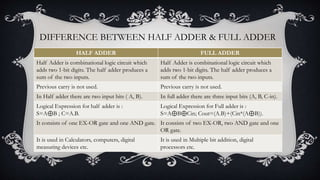1 de 16
Anúncio

1. HALF AND FULL ADDER D.C.Dharun Mughilan
2. WHAT IS AN ADDER? An Adder is a device that can add two binary digits. It is a type of digital circuit that performs the operation of additions of two number. It is mainly designed for the addition of binary number, but they can be used in various other applications like binary code decimal, address decoding, table index calculation, etc. There are two types of Adder. One is Half Adder, and another one is known as Full Adder.
3. Let us first take a look at the addition of single bits. 0 + 0 = 0 0 + 1 = 1 1 + 0 = 1 1 + 1 = 10 WHY WE NEED ADDERS?
4. These are the least possible single bit combinations. But the result for 1 + 1 =10. This problem can be solved with the help of an EX-OR gate. The sum results can be re-written as a 2-bit output. Thus the above combination can be written as: 0 + 0 = 00 0 + 1 = 01 1 + 0 = 01 1 + 1 = 10 Here the output “1” of “10” becomes the carry-out. SUM is the normal output and the CARRY is the carry-out. For this purpose we are using adders.
5. HALF ADDER  There are two inputs and two outputs in a Half Adder. Inputs are named as A and B, and the outputs are named as Sum (S) and Carry (C). The Sum is X-OR of the input A and B. Carry is AND of the input A and B. With the help of half adder, one can design a circuit that is capable of performing simple addition with the help of logic gates.
6. THE HALF ADDER CIRCUIT: 
7. TRUTH TABLE OF HALF ADDER INPUT OUTPUT A B SUM CARRY 0 0 0 0 0 1 1 0 1 0 1 0 1 1 0 1
8. From the truth table of the half adder we can see that the SUM (S) output is the result of the Exclusive-OR gate and the Carry- out (Cout) is the result of the AND gate. Then the Boolean expression for a half adder is as follows: For the SUM bit: 𝐴𝐵 + 𝐴𝐵 = 𝐴 𝑋𝑂𝑅 𝐵 = 𝐴⨁𝐵 For the CARRY bit: 𝐴 𝐴𝑁𝐷 𝐵 = 𝐴. 𝐵